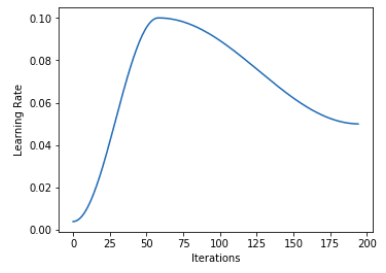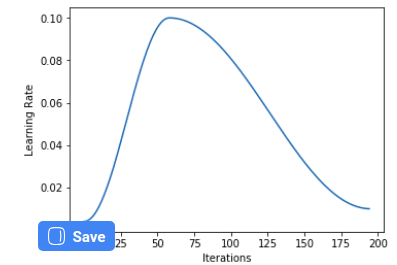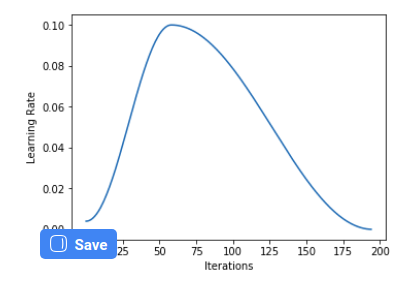# Shedding some light about LR management in fastai

There are a lot of unanswered questions, here on the forum, about almost every aspect related to the LR and its management in fastai.

Let’s start talking about the 1-cycle scheduler, which appears to be the most effective scheduling policy when it comes to LR management.

As Sylvain Gugger explains in his epic summary of Smith’s papers, one should do a few epochs at the end of the cycle, allowing the LR to go well below the minimum (which, by default, is 1/25 of the maximum in fastai), for the last few epochs. This allows us to go deeper in the minimum we got ourselves into.

AFAIK (and after a quick search) no one ever asked how to set this.
But then I noticed there is a promising `final_div` argument for the OneCycleScheduler callback.

And indeed it seems it should do the trick. But then, if you call `learn.recorder.plot_lr()` you can observe that the final epochs are performed with a LR well below the minimum even if you leave `final_div` to its default `None`. As a matter of fact, I noticed that the plot is the same no matter how you set `final_div`
Another interesting thing would be to set the length of these final epochs.

Question 1a: How can I set the LR for the final part?

Question 1b: How I can set the length of the final part?

Let’s talk a bit about `pct_start`. Smith suggests to spend more or less the same number of epochs both for the ascending and the descending part of the cycle. Still, we have a default of 0.3 for `pct_start`.

Question 2: Why did you choose to do fewer epochs for the ascending part? Furthermore, can you mention some cases when it would be advisable to do the contrary? E.g. here and there over the notebooks you do `0.1` or `0.9`, but I was unable to catch a pattern of usage.

Now, regarding the other stuff. We can pass a slice to `fit_one_cycle()`, or we can pass `max_lr=X`, or we can even pass `max_lr=slice(X,Y)`.

As far as I understand, there is no point in passing a slice unless we are working upon an unfrozen model. That is, `slice(X,Y)` sets the maximum LR to X for the early group, Y for the last group, and something in the middle for the central group (is this correct?).

`max_lr`, on the other hand, makes me scratch my head. The maximum LR should be the value we pass directly to `fit_one_cycle()`. That is, a call to `fit_one_cycle(10, 1e-3) should be exactly the same as`fit_one_cycle(10, max_lr=1e-3). So max_lr seems to be completely pointless.

Question 3: how does one make use of `max_lr`?

Last but not least: how to construe a plot like this:

Such a monstrosity comes from lesson 3 (segmentation).

As you may see, we don’t have any segment of negative slope (apart from a slight hint of it around 1e-5). Here is what Jeremy did:

`lrs = slice(1e-5,lr/5)`

`lr` here was `1e-2`. So, surprisingly enough, he is selecting `2e-3` as his maximum LR, and you can clearly see that at that point the loss is already starting to badly blow up.

That’s all. Thanks!

1 Like

I am not part of the fastai development, so you can add, ‘I think’ in front of all statements.

Why default is 1/25 of max?
From the report

There are several ways
one can choose the minimum learning rate bound: (1) a factor of 3 or 4 less than the maximum
bound, (2) a factor of 10 or 20 less than the maximum bound if only one cycle is used, (3) by a short
test of hundreds of iterations with a few initial learning rates and pick the largest one that allows
convergence to begin without signs of overfitting

So fastai is following the first approach by default.

How to set the final minimum value of learning rate?
final_div is the argument you need for this. For larger values, the difference is hard to notice, but when you use smaller values for final_div you can see the difference.
This is with final_div=2This is with final_div=10This is with defaultFrom the figures, we can say that final_div does control the minimum value. Also, in the source code we are dividing th max_lr by final_div, to set the minimum value of lr.
If you do not specify the value of final_div, a default of 1e4 is assigned to it.

How can I set the LR for the final part?
By using final_div

How I can set the length of the final part?
This is bit tricky. You might have seen the linear cycle for LR in most of the cases. But fastai uses cosine by default. So for cosine, there is not much sense of specifying the length of the final part, as it only depends on the value of the max_lr and min_lr. Now if you want to use other annealing functions(which you should not), then most probably you will have to modify this line in the source code.

I have actually forgotten that if we can set the annealing function by some argument or not. But in the source code when Scheduler is called it is given annealing_cos.

Why fewer epochs(iterations) for start?
It again depends on the annealing function you are using. So our main aim is to train our model on high value of learning rate for a longer time, but we have to train it for smaller values also.

Now if you see the plot of learning rate that I showed above, after reaching the maximum, learning rate is not decreasing rapidly, instead, it kind of decreases slowly, which king of displays training at high learning rate for longer. Now if the value of pct_start would have been 0.5 you can argue that we would not be able to train for smaller learning rate for more epochs. As in the end, in order to come with a stable solution, we have to train at smaller learning rates. Value of 0.3 kind of balances.

But again if you use linear annealing, you would have to change pct_start.

How to use the plot in Question 3?
Try with lower start_lr and more n_iter. If we have to comment on this graph, then it shows it has already trained and you have to train it at small learning rate 1e-7. I don’t know about 1e-5. I would check for the valid loss to decide on the final value.

slice
For the slice part, even I need some clarification. But source code for lr_range gives quite an idea, as in fit_one_cycle, lr_range is initially called to get value of learning rate.

``````def lr_range(self, lr:Union[float,slice])->np.ndarray:
"Build differential learning rates from `lr`."
if not isinstance(lr,slice): return lr
if lr.start: res = even_mults(lr.start, lr.stop, len(self.layer_groups))
else: res = [lr.stop/10]*(len(self.layer_groups)-1) + [lr.stop]
return np.array(res)
``````

6 Likes

I’ve been looking at this particular problem too, specifically around the slice, and how fit one cycle uses it.

From what I’ve seen in the code and docs, if we supply a slice to fit_one_cycle, discriminative learning rates are applied to layer groups. So I think if you setup a cnn_learner with a pre-trained network, you would get 2 layer groups by default. Assuming we have an unfrozen network, if you passed a slice to max_lr with two values, you would get one learning rate applied to the first layer group (the pre-trained layers) and another learning rate to the second layer group of fully connected layers.

So from this a few questions that I have:

1. Is what I just said correct? And if so, I assume the fit_one_cycle method varies the learning rate automatically according to the 1cycle policy, where it takes a max learning rate, and starts from a minimum value of max_lr/div_factor (I read this here: https://docs.fast.ai/callbacks.one_cycle.html)
2. If this is how fit_one_cycle operates, and it determines the minimum learning rate itself, how then do the learning rates in the slice passed to max_lr get used in each layer group by the 1cycle policy? Does:
a) fit_one_cycle operate on each layer group and apply one learning rate cycle for each group using the learning rate it obtained from its portion of the slice as its maximum? Or:
b) Does fit_one_cycle work across all of the layers, using a single learning rate for its max value. And if this is the case, how then does the slice get used?

Hope that all makes sense and doesn’t sound like nonsense.

1 Like

But the whole point of `final_div` would be to make some epochs with a very small learning rate. If you look at Gugger’s summary, he recommends something like one hundredth of the minimum. That is (leaving everything at default) 1/25 * 1/100. If you pass a final div of 2500, the plot should actually show the graph touching the line y=0, but this does not seem to happen.

Also, from your example plot with final div = 2, you can see it alters the whole cycle, not just the final part. Looking at the typical linear example, the final part is the line segment characterized by a slightly smaller negative slope:

The main cycle is the part of the graph above the line y=0.001, the final part is the one below that line. Setting such part should not alter the rest of the cycle.

I would not dare to tag Jeremy, but since he liked the answer, I would just ask him for clarification particularly about such matters.

Mh, why not? You can safely glue a cosine (in fact, since it starts from ~0, I would have called it sine annealing) with another function… It would be continuous nonetheless.

Just another thing: what is the point of having `max_lr`? It doesn’t seem to do anything useful…

I may be wrong, but I think that as you unfreeze the network, it get splitted, by default in three layer groups: the first half of the body, the last part of it, and the head. If you pass `slice(a,b)`, you will get `a` applied to the 1st part of the body, `b` to the head, and something in the middle to the 2nd part of the body. Indeed, rather than slice(), you can try and pass a list like this: `[a,c,b]`, a-la-fastai 0.7. It’ll work.

Correct, but don’t confuse the “variation” of LR for the 1-cycle policy with differential learning rates. They are very different concepts, and as you train an unfrozen network, both of them are applied.
Suppose you start fit_one_cycle() upon an unfrozen net, just specifying a learning rate of `lr=1`, and a slice like `(lr/a,lr)`. What follows will happen:

• It will do a cycle where the learning rate for the head varies between 1/25 and 1.
• The learning rate for the first group of the body will go between 1/25a and 1/a.
• The learning rate for the central group will vary accordingly within some middle ground. Looking at the code above, if layer_groups>3, the array will be spaced in proportion, unless you pass it explicitly.

Not at all!1 Like

Maybe @jeremy can help clarify this. Earlier when fastai used the linear cycle, it would create 3 scheds, for the 3 lines that we see in the graph.

Now fastai uses cosine annealing and it has only 2 phases.

``````self.phases = ((a1, annealing_cos), (a2, annealing_cos))
self.lr_scheds = self.steps((low_lr, self.lr_max), (self.lr_max, self.lr_max/self.final_div))
``````

For setting the minimum learning rate, it is set using `self.lr_max/self.final_div`, which shows that final_div is responsible for the minimum learning rate.

The above code can help you with this. We are only creating 2 scheds, which is different from linear cycle where we would create 3 cycle and only in the last sched we would use final_div, to get min_lr/100.

annealing_cos is not just taking the cosine of the value. See the code

``````def annealing_cos(start:Number, end:Number, pct:float)->Number:
"Cosine anneal from `start` to `end` as pct goes from 0.0 to 1.0."
cos_out = np.cos(np.pi * pct) + 1
return end + (start-end)/2 * cos_out
``````

We need max_lr, as we have to specify the maximum learning rate that our model would train. If we do not specify it, how would we set the maximum value of learning rate for our model.

``````low_lr = self.lr_max/self.div_factor
``````

Yes, when usingpretrained models we do get 3 groups

``````learn = cnn_learner(data, models.resnet18)
len(learn.layer_groups)
# 3
``````

Yes it starts from max_lr/div_factor -> max_lr and then max_lr->max_lr/final_div

What happens when we pass slice as max_lr?
So when we use lr_range and a slice is passed, we get np.ndarray as our value of max_lr which we pass to OneCycleScheduler callback.
In short, onecyclescheduler uses arrays where each element refers to the corresponding layer group. Check this code

``````max_lr = learn.lr_range(lr=slice(0.01, 0.1))
max_lr
# array([0.01    , 0.031623, 0.1     ])
low_lr = max_lr/25. # div_factor=25.
# array([0.0004  , 0.001265, 0.004   ])

min_lr = max_lr/(25.*1e4)
# So we get low_lr for each group and same for max_lr
``````
``````phases = ((2, annealing_cos), (3, annealing_cos))

def steps(*steps_cfg):
for (step, (n_iter, func)) in zip(steps_cfg, phases):
print(f'Step: {step}')
print(f'n_iter: {n_iter}')
print(f'func: {func}')
# Step: (array([0.0004  , 0.001265, 0.004   ]), array([0.01    , 0.031623, 0.1     ]))
# n_iter: 2
# func: <function annealing_cos at 0x7f95e18c2158>
# Step: (array([0.01    , 0.031623, 0.1     ]), array([4.000000e-08, 1.264911e-07, 4.000000e-07]))
# n_iter: 3
# func: <function annealing_cos at 0x7f95e18c2158>
``````

So Onecycle uses arrays representing learning rate for each group and when we have to update step, the required learning rate is used for that group.

2 Likes

Ok, thanks. I now understand how fastai chose to implement the 1-c policy. Still if I manually add a “final phase” (using `start_epoch`, so it attaches almost continuously to the previous cosine), going from my minimum lr to a fraction of it, I observe improvements. Slight, granted, but better than a vanilla 1-c.

We set it by just passing a LR. Indeed, it is the dafault. This will be the value which gets divided by 25 (or whatever you pass as div factor). And indeed in your code fragment:

``````low_lr = self.lr_max/self.div_factor
``````

`lr_max` is just the lr, if we don’t pass a `max_lr`.

Note: you steps-printing code is instructive, nonetheless! Add the loss to it, and you’ll almost get a LR finder for the other groups as wellI saw your earlier reply and it all looks spot-on to me!

2 Likes

Kushaj was very clear in explaining the 1-c implementation by fastai. Nonetheless, leaving apart `max_lr` which seems to be kind of redundant (or maybe placed there for future use), I still don’t understand why did you choose 2e-3 as your maximum lr given that graph.

Thank you everyone! I think I’m a whole lot clear on all of that now! I’ve been trying to figure this out for a few days now and learning about 1cycle policy as well so its good to get some clarity finallyI was looking at learn.model and visually trying to see the layer groups and assumed from that, that there were 2 layer groups instead of 3, but I was probably just interpreting that incorrectly. I suppose a good way to have gone about things would be to plot the learning rate during a fit_one_cycle training run to see how the LR changes throughout the layer groups. I’ll try get a plot of that up here as well maybe to add to the discussion.1 Like